# 10 Important Compound Interest Tricks

### Question 1.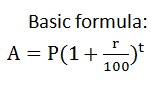Example: P=20000 Rs. , r= 10%. t= 2 years, A=?
Solution: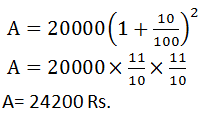### Question 2.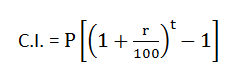Example: What will be the compound interest on Rs. 12000 after 3 years at the rate of 10% per annum?
Solution:### Question 3.

If a sum of money becomes n1 times itself at compound interest in t1 years then after t2 sum will be:Example: A sum of money becomes double itself in 5 years at compound interest. In how many years will it become 8 times of itself?
Solution:### Question 4.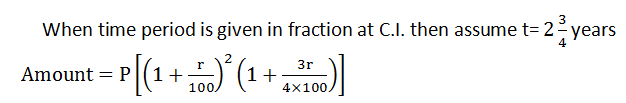Example: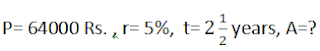Solution:### Question 5.

Calculation of instalment for 2 years:
Convert r% into fraction (a/b)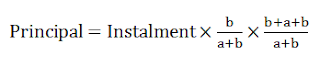Example: A man borrowed Rs.8,400 at 10% p.a. CI. He pays equal annual instalment and clear off his debts in 2 yrs. Find the instalment?
Solution:### Question 6.

Calculation of instalment for 3 years:
Convert r% into fraction (a/b)Example: A man borrowed Rs.397950 at 6% p.a. CI. He pays equal annual instalment and clear off his debts in 3 yrs. Find the instalment.
Solution:### Question 7.

If the difference between C.I. and S.I. for 2 years is given, then Principal:Example:  If the difference between S.I. and C.I. for 2 years on certain money is 4 Rs. The rate of interest is 8% per annum then find the money.
Solution:### Question 8.

If difference between C.I. and S.I. for 3 years is given, then Principal: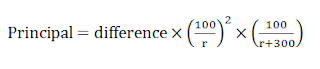Example:  If the difference between S.I. and C.I. for 3 years on certain money is 31 Rs. The rate of interest is 10% per annum then find the money.
Solution:### Question 9.

A sum of money invested at compound interest amounts to 800Rs. in 3 years and Rs. 882 in 5 years. What is the rate of interest?
Solution:

### Question 10.

When r% is given p.a. and CI has to be calculated half-yearly or quarterly basis:

Yearly factors r% (per annum) Time (n years)
Half yearly 6 months=
(6/12)=1/2
Factor ×r%= r/2% 2n
Quarterly 3 months= (3/12)=1/4 1/4 ×r% = r/4% 4n
9 months 9 months= (9/12)=3/4 3/4× r%= 3r/4% 4n/3
8 months 8 months= (8/12)= 2/3 2/3× r%= 2r/3% 3n/2